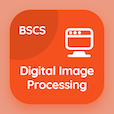Online CS Degree Courses

Digital Image Processing MCQs

Digital Image Processing MCQ - Topics

Practice Basic Intensity Transformations Functions Multiple Choice Questions (MCQ Quiz), Basic Intensity Transformations Functions quiz answers PDF to study digital image processing course for digital image processing online classes. Intensity Transformation and Spatial Filtering Multiple Choice Questions and Answers (MCQs), Basic Intensity Transformations Functions quiz questions for accelerated computer science degree online. Basic Intensity Transformations Functions Book PDF: smoothing spatial filters, histogram equalization, power law transformation test prep for computer and information science.

"The values of pixels in image processing are related by the expression" MCQ PDF: basic intensity transformations functions App APK with s = tr, s = (r), r = t(s), and t = sr choices for accelerated computer science degree online. Learn basic intensity transformations functions quiz questions for merit scholarship test and certificate programs for bachelor's degree in computer science.

## MCQ on Basic Intensity Transformations Functions Quiz

MCQ: The values of pixels in image processing are related by the expression

s = Tr
s = (r)
r = T(s)
T = sr

MCQ: In the expression s = Tr, T

copy the pixel values
replace the pixel values
enhance the pixel values
maps the pixel values

MCQ: The simplest image processing technique is

spatial transformation
intensity transformation
coordinates transformation
domain transformation

### More Quizzes on Digital Image Processing Book